Next: Dispersion Up: Geometric Optics Previous: Law of Refraction

## Total Internal Reflection

An interesting effect known as total internal reflection can occur when light attempts to move from a medium having a given refractive index to a medium having a lower refractive index. Suppose that light crosses an interface from medium 1 to medium 2, where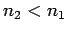. According to Snell's law,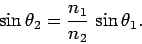(348)

Since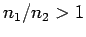, it follows that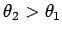. For relatively small angles of incidence, part of the light is refracted into the less optically dense medium, and part is reflected (there is always some reflection at an interface). When the angle of incidence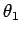is such that the angle of refraction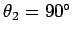, the refracted ray runs along the interface between the two media. This particular angle of incidence is called the critical angle,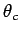. For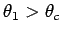, there is no refracted ray. Instead, all of the light incident on the interface is reflected--see Fig. 59. This effect is called total internal reflection, and occurs whenever the angle of incidence exceeds the critical angle. Now when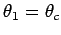, we have, and so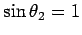. It follows from Eq. (348) that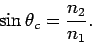(349)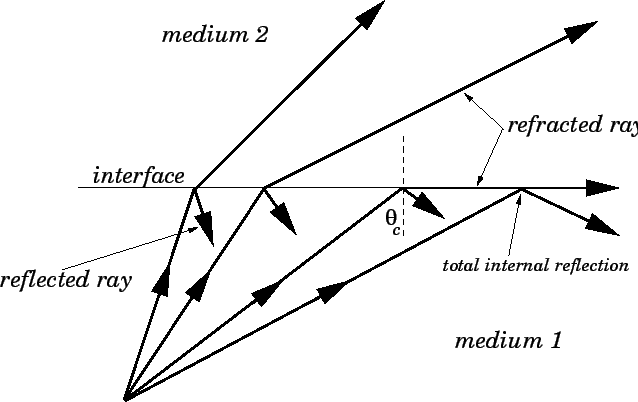Consider a fish (or a diver) swimming in a clear pond. As Fig. 60 makes clear, if the fish looks upwards it sees the sky, but if it looks at too large an angle to the vertical it sees the bottom of the pond reflected on the surface of the water. The critical angle to the vertical at which the fish first sees the reflection of the bottom of the pond is, of course, equal to the critical anglefor total internal reflection at an air-water interface. From Eq. (349), this critical angle is given by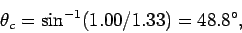(350)

since the refractive index of air is approximately unity, and the refractive index of water is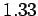.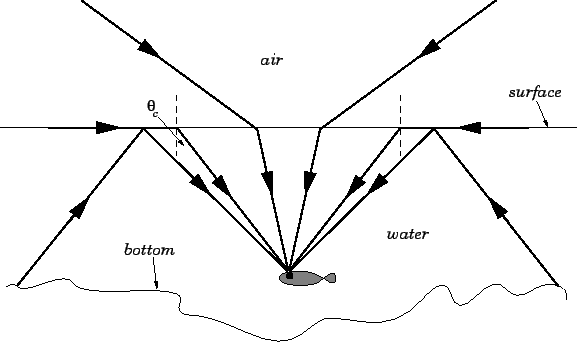When total internal reflection occurs at an interface the interface in question acts as a perfect reflector. This allows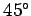crown glass prisms to be used, in place of mirrors, to reflect light in binoculars. This is illustrated in Fig. 61. The angles of incidence on the sides of the prism are all, which is greater than the critical angle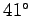for crown glass (at an air-glass interface).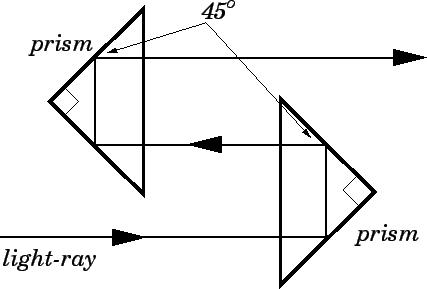Diamonds, for which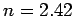, have a critical anglewhich is only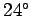. The facets on a diamond are cut in such a manner that much of the incident light on the diamond is reflected many times by successive total internal reflections before it escapes. This effect gives rise to the characteristic sparkling of cut diamonds.

Total internal reflection enables light to be transmitted inside thin glass fibers. The light is internally reflected off the sides of the fiber, and, therefore, follows the path of the fiber. Light can actually be transmitted around corners using a glass fiber, provided that the bends in the fiber are not too sharp, so that the light always strikes the sides of the fiber at angles greater than the critical angle. The whole field of fiber optics, with its many useful applications, is based on this effect.Next: Dispersion Up: Geometric Optics Previous: Law of Refraction
Richard Fitzpatrick 2007-07-14